# HTF PO3 Indicator For ThinkOrSwim

#### FutureTony

##### Well-known member
VIP
For those of you familiar with the ICT Power of 3 concept, this might be an indicator you would like. In a nutshell, PO3 is a concept that identifies Accumulation, Manipulation and Distribution on a smaller timeframe in the context of a higher timeframe candle. If you consider a bullish candle, there is an opening price, a wick below and then a move higher. The idea here is we want to be buyers below the open when we anticipate bullish price action. And obviously vice versa when we anticipate bearish price action.

Okay, on to the indicator. This indicator will display a daily (or other timeframe) candle to the right of your chart as a reference point for you. You must have your chart set with at least 4 bars in the expansion area for this indicator to work. Optional settings include; showing bubbles for the high/low/open of the higher timeframe candle, drawing horizontal dashes for the highs and lows and labels that display OHLC plus current range. There is also an option to show the opening price as the 'midnight open' vs the standard daily open. Again, this is an ICT concept that may or may not be of interest to you. You can adjust the color of the candles in the global settings for the indicator.

Additional inspiration for the display of this indicator comes from This TradingView indicator by @toodegrees.

You could also set this up to show a monthly candle on a daily chart, etc. This chart uses the unsupported AddChart feature for the wicks. As such, there can be some weirdness with the wick placement on different timeframes. For this reason, I've added a 'wick offset' option. Normally this should just be left at 1 but you can try to adjust it up or down(removes wick at zero) to accommodate.

As always, let me know what you think or if you have other ideas. The image below shows a 4hr candle on a 3m chart. I like this both with the 4hr and daily candles. YMMV.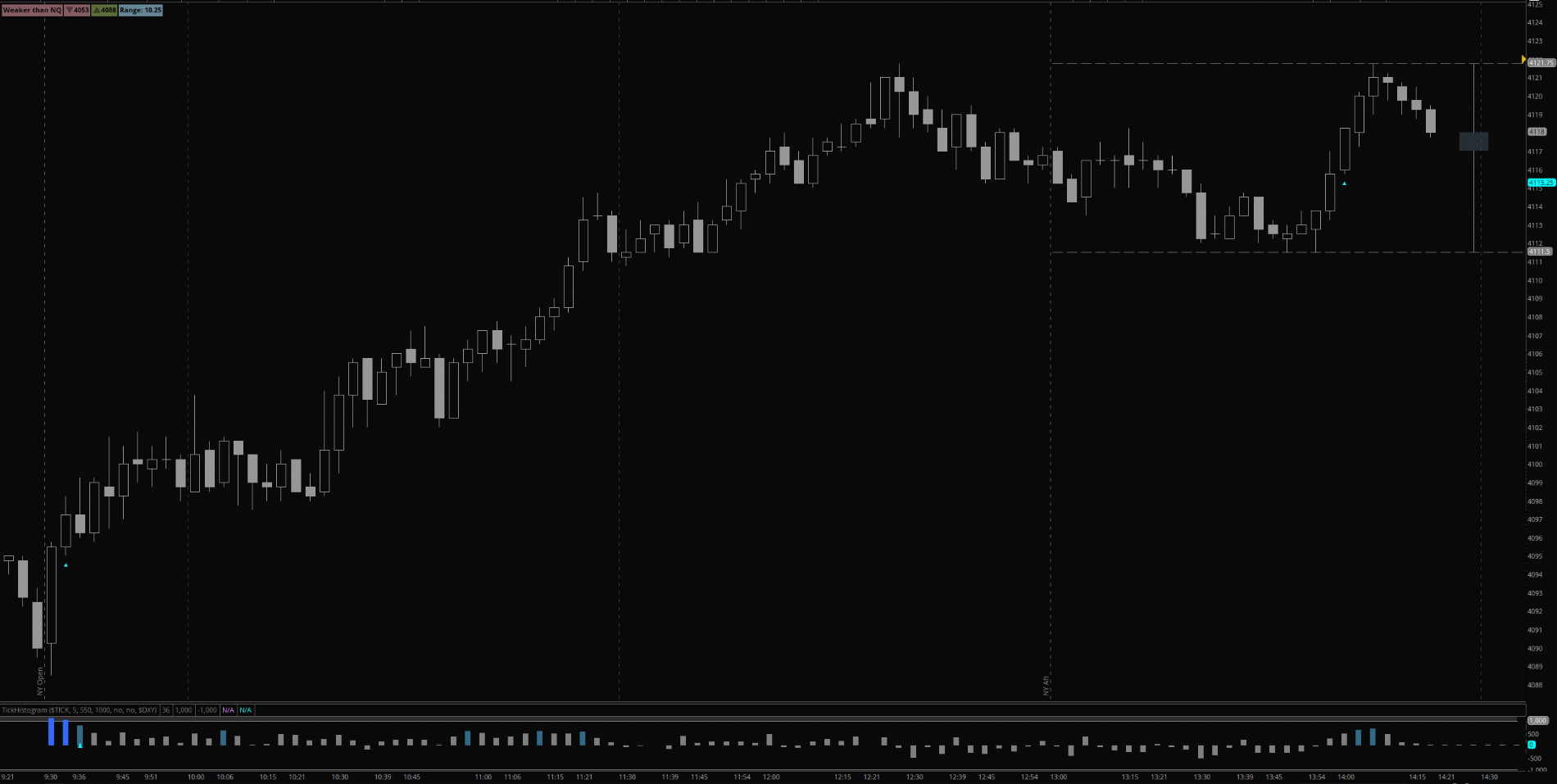Ruby:
``````#hint: <b>Intro</b> \n This study displays a higher timeframe candle to the right of the chart in the expansion area. \n <li>Typically used for showing PO3 for daily chart</li> <li>You must have an expansion area of 4 or greater set for this to work</li>
#hint useMidnight: <b>Only</b> for Day Aggregation.

# Created by @tony_futures inspired by the ICT Power of 3 concept

input htf = AggregationPeriod.DAY;
input useMidnight = no;
def midnight = 0000;
def isMidnight = secondsFromTime(midnight) == 0;
def midPrice = if isMidnight then open else midPrice;
def xO = if useMidnight then midPrice else open(period = htf);
def xH = high(period = htf);
def xL = low(period = htf);
def xC = close(period = htf);

input wickOffset = 1;

def exp2 = (isNaN(close[wickOffset]) OR isNaN(close[wickOffset+1]) OR isNaN(close[wickOffset+2])) and !isNan(close[wickOffset+3]);
def expMid = isNaN(close[wickOffset+1]) and !isNaN(close[wickOffset+2]);
def expEnd = isNaN(close[wickOffset+3]) and !isNaN(close[wickOffset+4]);
def NaN = Double.NaN;

def isUp = xo < xc;
def isDn = xo > xc;
def isDoji = xO == xC;

# Global Color setup
DefineGlobalColor("greenCandle", CreateColor(103, 135, 151));
DefineGlobalColor("redCandle", CreateColor(136, 93, 100));
DefineGlobalColor("wick", Color.GRAY);

AddCloud( if exp2 and isUp then xC else NaN, xO, GlobalColor("greenCandle"), GlobalColor("redCandle"));
AddCloud( if exp2 and isDn then xO else NaN, xC, GlobalColor("redCandle"), GlobalColor("redCandle"));

plot dojiWick = if isDoji and exp2 then xO else NaN;
dojiWick.setDefaultColor(GlobalColor("wick"));

def wickHigh = if isUp then xC else xO;
def wickLow = if isDn then xC else xO;
AddChart(if expMid then xH else NaN, if expMid then wickHigh else Double.NaN,  NaN,  NaN, ChartType.BAR, GlobalColor("wick"));
AddChart(if expMid then xL else NaN, if expMid then wickLow else Double.NaN,  NaN,  NaN, ChartType.BAR, GlobalColor("wick"));

input showTimeFrameBubble = no;
def tf = htf/60000;
AddChartBubble(showTimeFrameBubble and expMid,xL,if tf == 1440 then "D" else if tf == 240 then "4h" else if tf == 120 then "2h" else if tf == 60 then "H" else AsText(tf),Color.WHITE,no);

input showBubbles = yes;
AddChartBubble(showBubbles and expEnd and isUp, xO, xO, GlobalColor("greenCandle"), no);
AddChartBubble(showBubbles and expEnd and isDn, xO, xO, GlobalColor("redCandle"), yes);
AddChartBubble(showBubbles and expEnd, xL, xL, if isUp then GlobalColor("greenCandle") else GlobalColor("redCandle"), no);
AddChartBubble(showBubbles and expEnd, xH, xH, if isUp then GlobalColor("greenCandle") else GlobalColor("redCandle"), yes);

input showLabels = no;
def candleRange = xH - xL;
AddLabel(showLabels,(if tf == 1440 then "D" else if tf == 240 then "4h" else if tf == 120 then "2h" else if tf == 60 then "H" else AsText(tf)) + " Range: " + candleRange, if isUp then GlobalColor("greenCandle") else if isDn then GlobalColor("redCandle") else GlobalColor("wick"));

input length = 14;
input averageType = AverageType.WILDERS;
def ATR = Round(MovingAverage(averageType, TrueRange(xH, xC, xL), length),1);

def xCL = close(period = htf)[-1];
def lastCandle = isNaN(xCL);

plot currentHigh = if showAdditionalLines and lastCandle then xH else NaN;
currentHigh.setPaintingStrategy(PaintingStrategy.DASHES);
currentHigh.setDefaultColor(GlobalColor("wick"));
currentHigh.DefineColor("Normal", GlobalColor("wick"));
currentHigh.DefineColor("Daily", GlobalColor("greenCandle"));
currentHigh.AssignValueColor(if tf == 1440 then currentHigh.Color("Daily") else currentHigh.Color("Normal"));

plot currentLow = if showAdditionalLines and lastCandle then xL else NaN;
currentLow.setPaintingStrategy(PaintingStrategy.DASHES);
currentLow.setDefaultColor(GlobalColor("wick"));
currentLow.DefineColor("Normal", GlobalColor("wick"));
currentLow.DefineColor("Daily", GlobalColor("redCandle"));
currentLow.AssignValueColor(if tf == 1440 then currentLow.Color("Daily") else currentLow.Color("Normal"));``````

Last edited:
•••SJP07, ShinJ, Intrinsic Knowledge and 5 others
•8Nick8 and FutureTony
For those of you familiar with the ICT Power of 3 concept, this might be an indicator you would like. In a nutshell, PO3 is a concept that identifies Accumulation, Manipulation and Distribution on a smaller timeframe in the context of a higher timeframe candle. If you consider a bullish candle, there is an opening price, a wick below and then a move higher. The idea here is we want to be buyers below the open when we anticipate bullish price action. And obviously vice versa when we anticipate bearish price action.

Okay, on to the indicator. This indicator will display a daily (or other timeframe) candle to the right of your chart as a reference point for you. You must have your chart set with at least 4 bars in the expansion area for this indicator to work. Optional settings include; showing bubbles for the high/low/open of the higher timeframe candle, drawing horizontal dashes for the highs and lows and labels that display OHLC plus current range. There is also an option to show the opening price as the 'midnight open' vs the standard daily open. Again, this is an ICT concept that may or may not be of interest to you. You can adjust the color of the candles in the global settings for the indicator.

Additional inspiration for the display of this indicator comes from This TradingView indicator by @toodegrees.

You could also set this up to show a monthly candle on a daily chart, etc. This chart uses the unsupported AddChart feature for the wicks. As such, there can be some weirdness with the wick placement on different timeframes. For this reason, I've added a 'wick offset' option. Normally this should just be left at 1 but you can try to adjust it up or down(removes wick at zero) to accommodate.

As always, let me know what you think or if you have other ideas. The image below shows a 4hr candle on a 3m chart. I like this both with the 4hr and daily candles. YMMV.Ruby:
``````#hint: <b>Intro</b> \n This study displays a higher timeframe candle to the right of the chart in the expansion area. \n <li>Typically used for showing PO3 for daily chart</li> <li>You must have an expansion area of 4 or greater set for this to work</li>
#hint useMidnight: <b>Only</b> for Day Aggregation.

# Created by @tony_futures inspired by the ICT Power of 3 concept

input htf = AggregationPeriod.DAY;
input useMidnight = no;
def midnight = 0000;
def isMidnight = secondsFromTime(midnight) == 0;
def midPrice = if isMidnight then open else midPrice;
def xO = if useMidnight then midPrice else open(period = htf);
def xH = high(period = htf);
def xL = low(period = htf);
def xC = close(period = htf);

input wickOffset = 1;

def exp2 = (isNaN(close) OR isNaN(close) OR isNaN(close)) and !isNan(close);
def expMid = isNaN(close[wickOffset+1]) and !isNaN(close[wickOffset+2]);
def expEnd = isNaN(close) and !isNaN(close);
def NaN = Double.NaN;

def isUp = xo < xc;
def isDn = xo > xc;
def isDoji = xO == xC;

# Global Color setup
DefineGlobalColor("greenCandle", CreateColor(103, 135, 151));
DefineGlobalColor("redCandle", CreateColor(136, 93, 100));
DefineGlobalColor("wick", Color.GRAY);

AddCloud( if exp2 and isUp then xC else NaN, xO, GlobalColor("greenCandle"), GlobalColor("redCandle"));
AddCloud( if exp2 and isDn then xO else NaN, xC, GlobalColor("redCandle"), GlobalColor("redCandle"));

plot dojiWick = if isDoji and exp2 then xO else NaN;
dojiWick.setDefaultColor(GlobalColor("wick"));

def wickHigh = if isUp then xC else xO;
def wickLow = if isDn then xC else xO;
AddChart(if expMid then xH else NaN, if expMid then wickHigh else Double.NaN,  NaN,  NaN, ChartType.BAR, GlobalColor("wick"));
AddChart(if expMid then xL else NaN, if expMid then wickLow else Double.NaN,  NaN,  NaN, ChartType.BAR, GlobalColor("wick"));

input showBubbles = yes;
AddChartBubble(showBubbles and expEnd and isUp, xO, xO, GlobalColor("greenCandle"), no);
AddChartBubble(showBubbles and expEnd and isDn, xO, xO, GlobalColor("redCandle"), yes);
AddChartBubble(showBubbles and expEnd, xL, xL, if isUp then GlobalColor("greenCandle") else GlobalColor("redCandle"), no);
AddChartBubble(showBubbles and expEnd, xH, xH, if isUp then GlobalColor("greenCandle") else GlobalColor("redCandle"), yes);

input showLabels = no;
def candleRange = xH - xL;
AddLabel(showLabels,"Range: " + candleRange, if isUp then GlobalColor("greenCandle") else if isDn then GlobalColor("redCandle") else GlobalColor("wick"));

def xCL = close(period = htf)[-1];
def lastCandle = isNaN(xCL);
#def candleChange = xO != xO;

plot currentHigh = if showAdditionalLines and lastCandle then xH else NaN;
currentHigh.setPaintingStrategy(PaintingStrategy.DASHES);
currentHigh.setDefaultColor(GlobalColor("wick"));

plot currentLow = if showAdditionalLines and lastCandle then xL else NaN;
currentLow.setPaintingStrategy(PaintingStrategy.DASHES);
currentLow.setDefaultColor(GlobalColor("wick"));``````
I've updated this one slightly. You can now add a second TF candle to your chart and I'm displaying the usual ATR for the higher timeframe candle in the labels. If you want to add a second timeframe, you need at least 7 bars in your expansion area: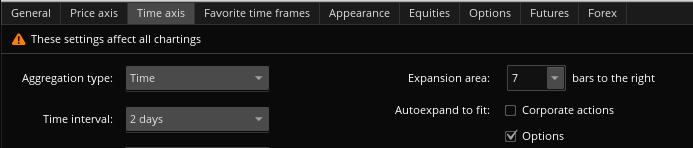And this is what a 3m chart looks like with a Daily and Hourly candle to the right: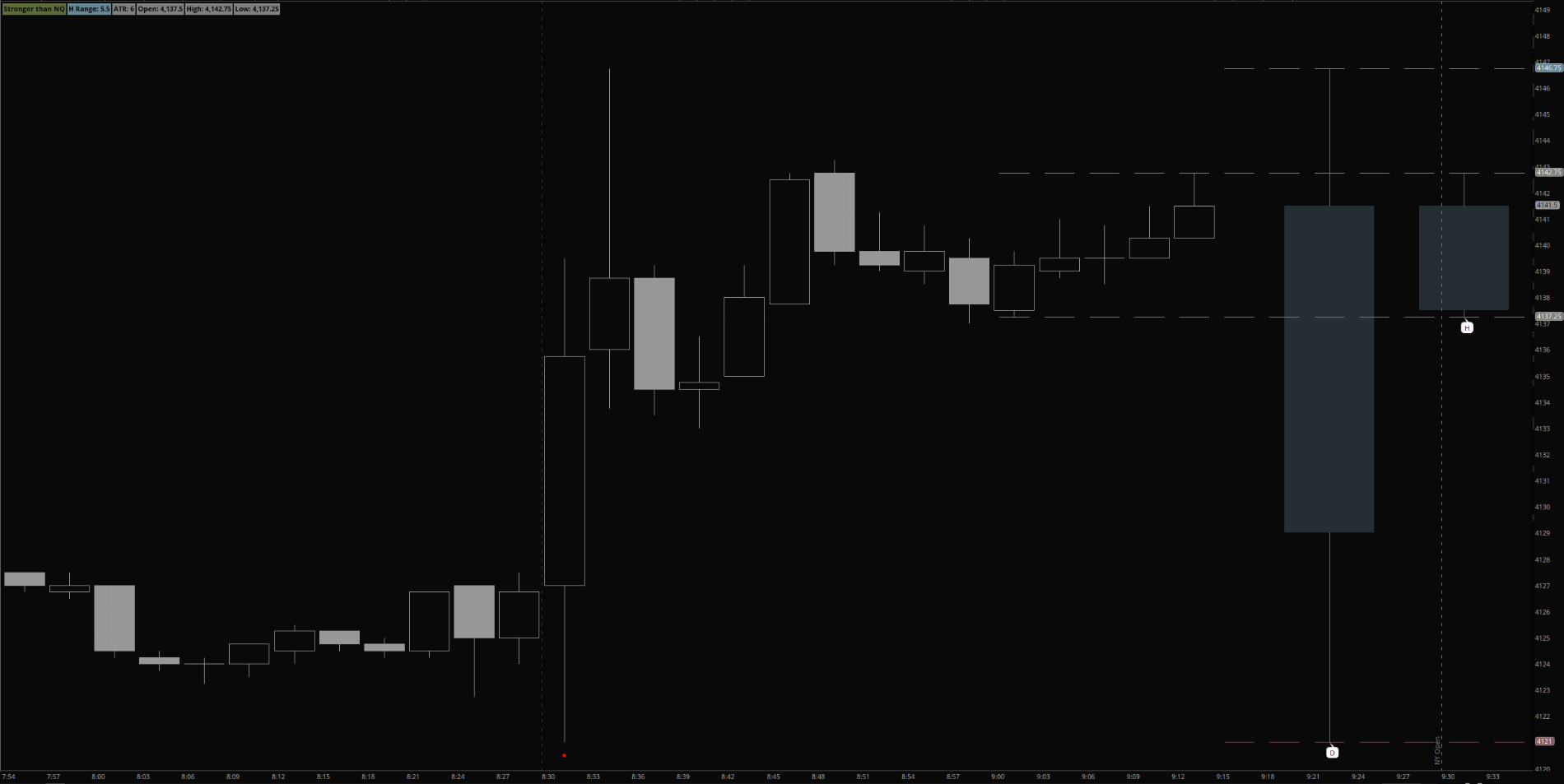In order to add the second candle, you must adjust the 'wickoffset' option to 4. Enjoy!

•ziko and BenTen
I've updated this one slightly. You can now add a second TF candle to your chart and I'm displaying the usual ATR for the higher timeframe candle in the labels. If you want to add a second timeframe, you need at least 7 bars in your expansion area:And this is what a 3m chart looks like with a Daily and Hourly candle to the right:In order to add the second candle, you must adjust the 'wickoffset' option to 4. Enjoy!
One more image to share from today's session. I find watching the larger timeframe candles helpful as they slow you down (as opposed to the smaller timeframe which can make you 'speed up'). Bullish 4hr candle and small retracement on the hourly into an area of interest. Took the long and watched the hourly flip back to bullish to close the day.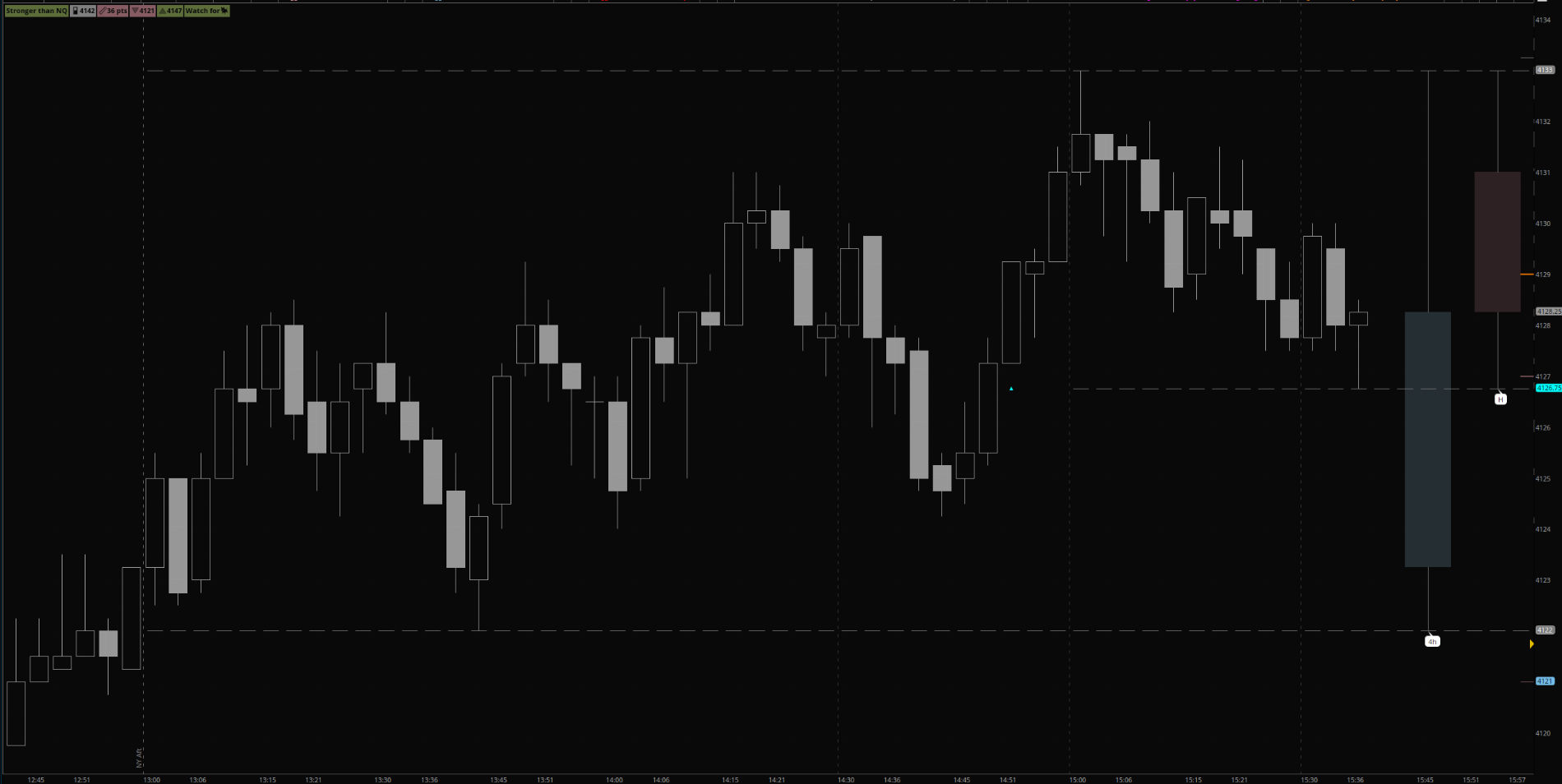••Helles, ziko, tenacity11 and 1 other person
For those of you familiar with the ICT Power of 3 concept, this might be an indicator you would like. In a nutshell, PO3 is a concept that identifies Accumulation, Manipulation and Distribution on a smaller timeframe in the context of a higher timeframe candle. If you consider a bullish candle, there is an opening price, a wick below and then a move higher. The idea here is we want to be buyers below the open when we anticipate bullish price action. And obviously vice versa when we anticipate bearish price action.

Okay, on to the indicator. This indicator will display a daily (or other timeframe) candle to the right of your chart as a reference point for you. You must have your chart set with at least 4 bars in the expansion area for this indicator to work. Optional settings include; showing bubbles for the high/low/open of the higher timeframe candle, drawing horizontal dashes for the highs and lows and labels that display OHLC plus current range. There is also an option to show the opening price as the 'midnight open' vs the standard daily open. Again, this is an ICT concept that may or may not be of interest to you. You can adjust the color of the candles in the global settings for the indicator.

Additional inspiration for the display of this indicator comes from This TradingView indicator by @toodegrees.

You could also set this up to show a monthly candle on a daily chart, etc. This chart uses the unsupported AddChart feature for the wicks. As such, there can be some weirdness with the wick placement on different timeframes. For this reason, I've added a 'wick offset' option. Normally this should just be left at 1 but you can try to adjust it up or down(removes wick at zero) to accommodate.

As always, let me know what you think or if you have other ideas. The image below shows a 4hr candle on a 3m chart. I like this both with the 4hr and daily candles. YMMV.Ruby:
``````#hint: <b>Intro</b> \n This study displays a higher timeframe candle to the right of the chart in the expansion area. \n <li>Typically used for showing PO3 for daily chart</li> <li>You must have an expansion area of 4 or greater set for this to work</li>
#hint useMidnight: <b>Only</b> for Day Aggregation.

# Created by @tony_futures inspired by the ICT Power of 3 concept

input htf = AggregationPeriod.DAY;
input useMidnight = no;
def midnight = 0000;
def isMidnight = secondsFromTime(midnight) == 0;
def midPrice = if isMidnight then open else midPrice;
def xO = if useMidnight then midPrice else open(period = htf);
def xH = high(period = htf);
def xL = low(period = htf);
def xC = close(period = htf);

input wickOffset = 1;

def exp2 = (isNaN(close[wickOffset]) OR isNaN(close[wickOffset+1]) OR isNaN(close[wickOffset+2])) and !isNan(close[wickOffset+3]);
def expMid = isNaN(close[wickOffset+1]) and !isNaN(close[wickOffset+2]);
def expEnd = isNaN(close[wickOffset+3]) and !isNaN(close[wickOffset+4]);
def NaN = Double.NaN;

def isUp = xo < xc;
def isDn = xo > xc;
def isDoji = xO == xC;

# Global Color setup
DefineGlobalColor("greenCandle", CreateColor(103, 135, 151));
DefineGlobalColor("redCandle", CreateColor(136, 93, 100));
DefineGlobalColor("wick", Color.GRAY);

AddCloud( if exp2 and isUp then xC else NaN, xO, GlobalColor("greenCandle"), GlobalColor("redCandle"));
AddCloud( if exp2 and isDn then xO else NaN, xC, GlobalColor("redCandle"), GlobalColor("redCandle"));

plot dojiWick = if isDoji and exp2 then xO else NaN;
dojiWick.setDefaultColor(GlobalColor("wick"));

def wickHigh = if isUp then xC else xO;
def wickLow = if isDn then xC else xO;
AddChart(if expMid then xH else NaN, if expMid then wickHigh else Double.NaN,  NaN,  NaN, ChartType.BAR, GlobalColor("wick"));
AddChart(if expMid then xL else NaN, if expMid then wickLow else Double.NaN,  NaN,  NaN, ChartType.BAR, GlobalColor("wick"));

input showTimeFrameBubble = no;
def tf = htf/60000;
AddChartBubble(showTimeFrameBubble and expMid,xL,if tf == 1440 then "D" else if tf == 240 then "4h" else if tf == 120 then "2h" else if tf == 60 then "H" else AsText(tf),Color.WHITE,no);

input showBubbles = yes;
AddChartBubble(showBubbles and expEnd and isUp, xO, xO, GlobalColor("greenCandle"), no);
AddChartBubble(showBubbles and expEnd and isDn, xO, xO, GlobalColor("redCandle"), yes);
AddChartBubble(showBubbles and expEnd, xL, xL, if isUp then GlobalColor("greenCandle") else GlobalColor("redCandle"), no);
AddChartBubble(showBubbles and expEnd, xH, xH, if isUp then GlobalColor("greenCandle") else GlobalColor("redCandle"), yes);

input showLabels = no;
def candleRange = xH - xL;
AddLabel(showLabels,(if tf == 1440 then "D" else if tf == 240 then "4h" else if tf == 120 then "2h" else if tf == 60 then "H" else AsText(tf)) + " Range: " + candleRange, if isUp then GlobalColor("greenCandle") else if isDn then GlobalColor("redCandle") else GlobalColor("wick"));

input length = 14;
input averageType = AverageType.WILDERS;
def ATR = Round(MovingAverage(averageType, TrueRange(xH, xC, xL), length),1);

def xCL = close(period = htf)[-1];
def lastCandle = isNaN(xCL);

plot currentHigh = if showAdditionalLines and lastCandle then xH else NaN;
currentHigh.setPaintingStrategy(PaintingStrategy.DASHES);
currentHigh.setDefaultColor(GlobalColor("wick"));
currentHigh.DefineColor("Normal", GlobalColor("wick"));
currentHigh.DefineColor("Daily", GlobalColor("greenCandle"));
currentHigh.AssignValueColor(if tf == 1440 then currentHigh.Color("Daily") else currentHigh.Color("Normal"));

plot currentLow = if showAdditionalLines and lastCandle then xL else NaN;
currentLow.setPaintingStrategy(PaintingStrategy.DASHES);
currentLow.setDefaultColor(GlobalColor("wick"));
currentLow.DefineColor("Normal", GlobalColor("wick"));
currentLow.DefineColor("Daily", GlobalColor("redCandle"));
currentLow.AssignValueColor(if tf == 1440 then currentLow.Color("Daily") else currentLow.Color("Normal"));``````
@FutureTony Hi bro, thank you for this script. As below image, somehow when I use daily TF and change to the input to "Weekly". (1) It does not show the bubbles chart. Additionally, (2) the wick is not in the centre of the candle. I did try to adjust the number for the "wickoffset" but it does not make the wick in the centre of the candle. Do you know why?

@FutureTony Hi bro, thank you for this script. As below image, somehow when I use daily TF and change to the input to "Weekly". (1) It does not show the bubbles chart. Additionally, (2) the wick is not in the centre of the candle. I did try to adjust the number for the "wickoffset" but it does not make the wick in the centre of the candle. Do you know why?
I will take a look at this today and try to figure out what is going on.

•Intrinsic Knowledge
@FutureTony Hi bro, thank you for this script. As below image, somehow when I use daily TF and change to the input to "Weekly". (1) It does not show the bubbles chart. Additionally, (2) the wick is not in the centre of the candle. I did try to adjust the number for the "wickoffset" but it does not make the wick in the centre of the candle. Do you know why?
Okay, I think I've figured it out. As we look 'in the future' on the charts, it gets confused when the next daily candle is going to start. So built in a little work around.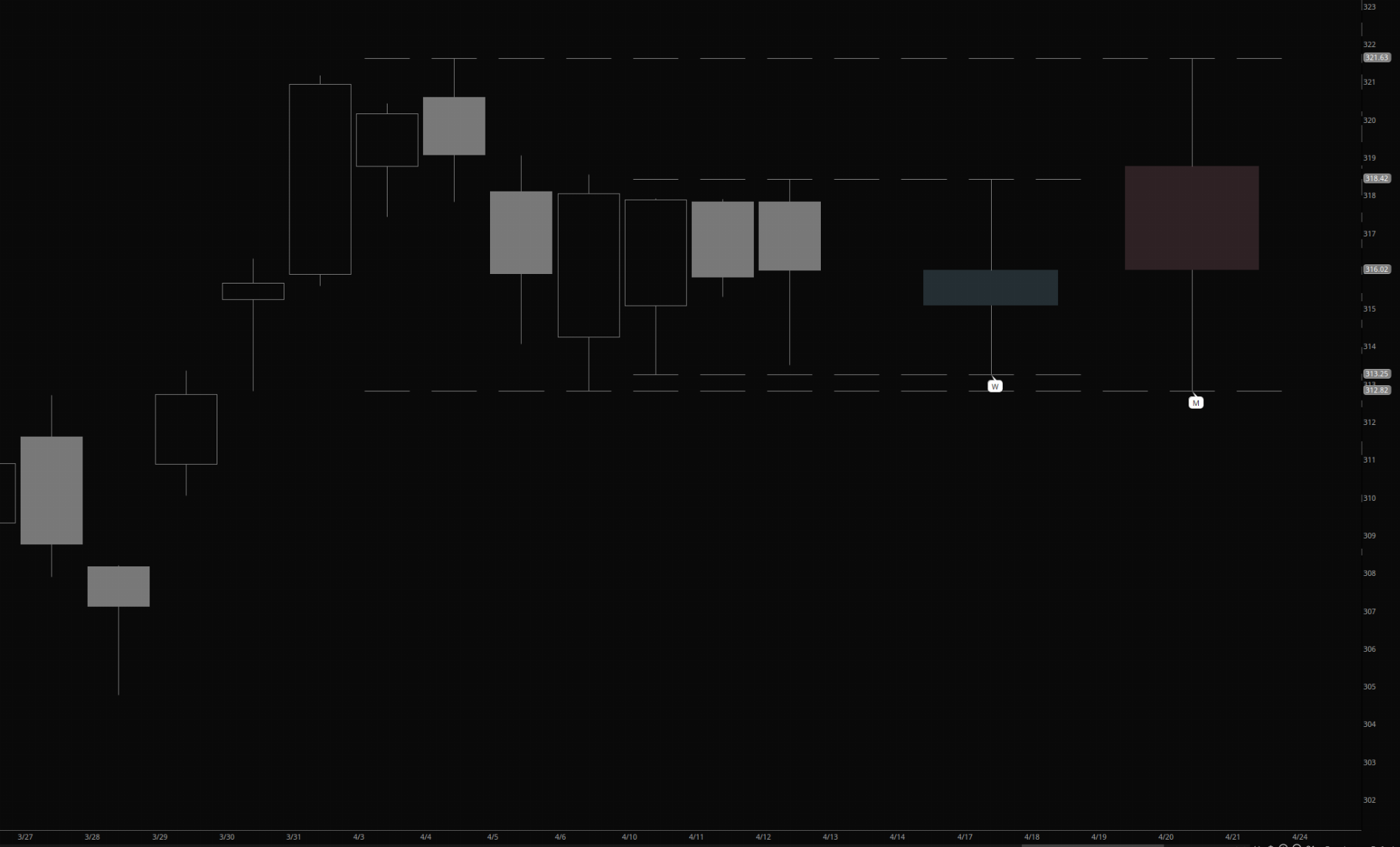I'm going to place a new version here as I haven't tested it quite enough to replace the original but wanted to get this out there to solve your issue:

Ruby:
``````#hint: <b>Intro</b> \n This study displays a higher timeframe candle to the right of the chart in the expansion area. \n <li>Typically used for showing PO3 for daily chart</li> <li>You must have an expansion area of 4 or greater set for this to work</li>
#hint useMidnight: <b>Only</b> for Day Aggregation.

# Created by @tony_futures inspired by the ICT Power of 3 concept

input htf = AggregationPeriod.DAY;
input useMidnight = no;
input candle = { default "First", "Second", "Third"};
def candleCount;

switch (candle)
{
case "First":
candleCount = 1;

case "Second":
candleCount = 4;
case "Third":
candleCount = 7;
}

def wickOffset = candleCount;
def midnight = 0000;
def isMidnight = secondsFromTime(midnight) == 0;
def midPrice = if isMidnight then open else midPrice;
def xO0 = if useMidnight then midPrice else open(period = htf);
def xH0 = high(period = htf);
def xL0 = low(period = htf);
def xC0 = close(period = htf);
def xH = if isNaN(xH0) and isNaN(xH0) then xH0 else if isNaN(xH0) then xH0 else xH0;
def xO = if isNaN(xO0) and isNaN(xO0[wickOffset]) and isNaN(xO0[wickOffset+1]) and isNaN(xO0[wickOffset+2]) and isNaN(xO0[wickOffset+3]) then xO0[wickOffset+4] else if isNaN(xO0) and isNaN(xO0[wickOffset]) and isNaN(xO0[wickOffset+1]) and isNaN(xO0[wickOffset+2]) then xO0[wickOffset+3] else  if isNaN(xO0) and isNaN(xO0[wickOffset]) and isNaN(xO0[wickOffset+1]) and isNaN(xO0[wickOffset+2]) then xO0[wickOffset+3] else if isNaN(xO0) and isNaN(xO0[wickOffset]) and isNaN(xO0[wickOffset+1]) then xO0[wickOffset+2] else if isNaN(xO0) and isNaN(xO0[wickOffset]) then xO0[wickOffset+1] else if isNaN(xO0) and !isNaN(xO0) then xO0[wickOffset] else xO0[wickOffset];
#def xC = if isNaN(xC0) then xC0 else xC0;
def xC = if isNaN(xC0) and isNaN(xC0) and isNaN(xC0) then xC0 else if isNaN(xC0) and isNaN(xC0) then xC0 else if isNaN(xC0) then xC0 else xC0;
def xL = if isNaN(xL0) and isNaN(xL0) and isNaN(xL0) then xL0 else if isNaN(xL0) and isNaN(xL0) then xL0 else if isNaN(xL0) then xL0 else xL0;

def exp2 = (isNaN(close[wickOffset]) OR isNaN(close[wickOffset+1]) OR isNaN(close[wickOffset+2])) and !isNan(close[wickOffset+3]);
def expMid = isNaN(close[wickOffset+1]) and !isNaN(close[wickOffset+2]);
def expEnd = isNaN(close[wickOffset+3]) and !isNaN(close[wickOffset+4]);
def expFinal = isNaN(close[wickoffset+3]);
def NaN = Double.NaN;

def isUp = xo < xc;
def isDn = xo > xc;
def isDoji = xO == xC;

# Global Color setup
DefineGlobalColor("greenCandle", CreateColor(103, 135, 151));
DefineGlobalColor("redCandle", CreateColor(136, 93, 100));
DefineGlobalColor("wick", Color.GRAY);

AddCloud( if exp2 and isUp then xC else NaN, xO, GlobalColor("greenCandle"), GlobalColor("redCandle"));
AddCloud( if exp2 and isDn then xO else NaN, xC, GlobalColor("redCandle"), GlobalColor("redCandle"));

plot dojiWick = if isDoji and exp2 then xO else NaN;
dojiWick.setDefaultColor(GlobalColor("wick"));

def wickHigh = if isUp then xC else xO;
def wickLow = if isDn then xC else xO;
AddChart(if expMid then xH else NaN, if expMid then wickHigh else Double.NaN,  NaN,  NaN, ChartType.BAR, GlobalColor("wick"));
AddChart(if expMid then xL else NaN, if expMid then wickLow else Double.NaN,  NaN,  NaN, ChartType.BAR, GlobalColor("wick"));

input showTimeFrameBubble = no;
def tf = htf/60000;
AddChartBubble(showTimeFrameBubble and expMid,xL,if tf == 43200 then "M" else if tf == 10080 then "W" else if tf == 1440 then "D" else if tf == 240 then "4h" else if tf == 120 then "2h" else if tf == 60 then "H" else Round(tf,0)+"",Color.WHITE,no);

input showBubbles = yes;
AddChartBubble(showBubbles and expEnd and isUp, xO, xO, GlobalColor("greenCandle"), no);
AddChartBubble(showBubbles and expEnd and isDn, xO, xO, GlobalColor("redCandle"), yes);
AddChartBubble(showBubbles and expEnd, xL, xL, if isUp then GlobalColor("greenCandle") else GlobalColor("redCandle"), no);
AddChartBubble(showBubbles and expEnd, xH, xH, if isUp then GlobalColor("greenCandle") else GlobalColor("redCandle"), yes);

input showLabels = no;
def candleRange = xH - xL;
AddLabel(showLabels,(if tf == 43200 then "M" else if tf == 10080 then "W" else if tf == 1440 then "D" else if tf == 240 then "4h" else if tf == 120 then "2h" else if tf == 60 then "H" else AsText(tf)) + " Range: " + candleRange, if isUp then GlobalColor("greenCandle") else if isDn then GlobalColor("redCandle") else GlobalColor("wick"));

input length = 14;
input averageType = AverageType.WILDERS;
def ATR = Round(MovingAverage(averageType, TrueRange(xH, xC, xL), length),1);

def xCL = close(period = htf)[-1];
def lastCandle = isNaN(xCL);

plot currentHigh = if showAdditionalLines and lastCandle and !expFinal then xH else NaN;
currentHigh.setPaintingStrategy(PaintingStrategy.DASHES);
currentHigh.setDefaultColor(GlobalColor("wick"));
currentHigh.DefineColor("Normal", GlobalColor("wick"));
currentHigh.DefineColor("Daily", GlobalColor("greenCandle"));
currentHigh.AssignValueColor(if tf == 1440 then currentHigh.Color("Daily") else currentHigh.Color("Normal"));

plot currentLow = if showAdditionalLines and lastCandle and !expFinal then xL else NaN;
currentLow.setPaintingStrategy(PaintingStrategy.DASHES);
currentLow.setDefaultColor(GlobalColor("wick"));
currentLow.DefineColor("Normal", GlobalColor("wick"));
currentLow.DefineColor("Daily", GlobalColor("redCandle"));
currentLow.AssignValueColor(if tf == 1440 then currentLow.Color("Daily") else currentLow.Color("Normal"));``````

Last edited:
Thanks bro. Cool!

@FutureTony Thanks for your time and efforts with this interesting indicator. I've tried installing both versions and I can't get the HTF candles to display...Any thoughts?

@FutureTony Thanks for your time and efforts with this interesting indicator. I've tried installing both versions and I can't get the HTF candles to display...Any thoughts?
What timeframes are you using for your chart and the indicator? I just made a change to the updated version - maybe try grabbing that one just in case and let me know how you are using it.

•MerryDay
@FutureTony Thanks for the swift reply and support...

What timeframes are you using for your chart and the indicator?

Indicator(Daily), per stated requirement, and Chart(10 min)...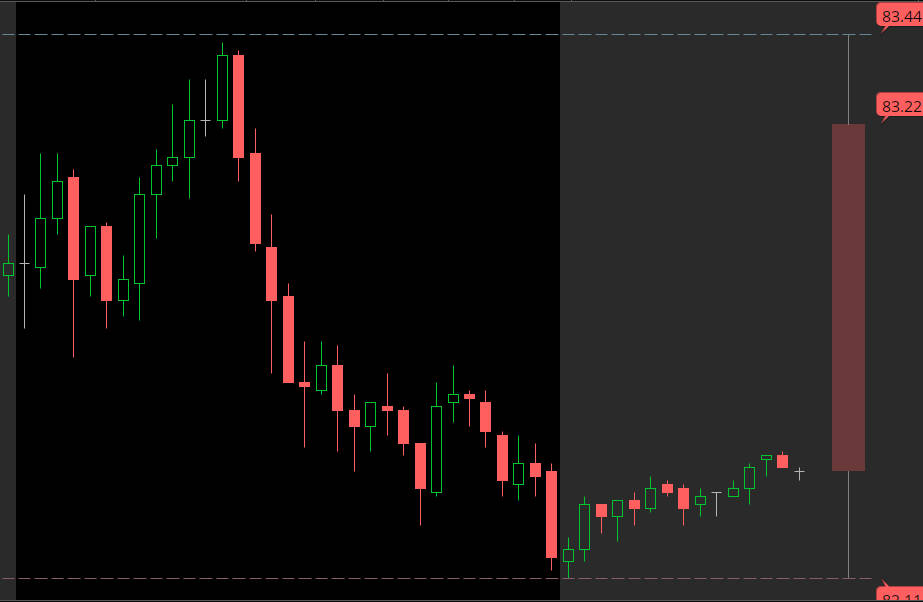Of course, now it works...LOL...but what about the second HTF candle, as illustrated in your screenshots?

Thanks again@FutureTony I got the second HTF candle working...

10 Min Chart...HTF Daily...HTF2 30 Min...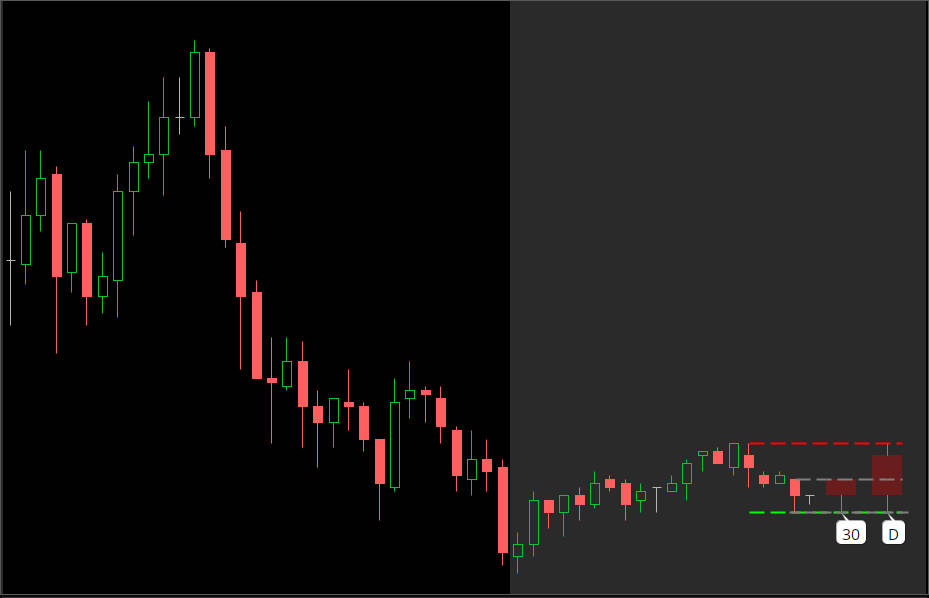Code:
``````# filename: ICT_Power_of_3_III_

#hint: <b>Intro</b> \n This study displays a higher timeframe candle to the right of the chart in the expansion area. \n <li>Typically used for showing PO3 for daily chart</li> <li>You must have an expansion area of 4 or greater set for this to work</li>
#hint useMidnight: <b>Only</b> for Day Aggregation.

# Created by @tony_futures inspired by the ICT Power of 3 concept

input htf = AggregationPeriod.DAY;
input htf2 = AggregationPeriod.THIRTY_MIN;

input useMidnight = no;
def midnight = 0000;
def isMidnight = secondsFromTime(midnight) == 0;
def midPrice = if isMidnight then open else midPrice;

# Global Color setup
DefineGlobalColor("greenCandle", CreateColor(0, 255, 0)); #(103, 135, 151));
DefineGlobalColor("redCandle", CreateColor(255, 0, 0)); #(136, 93, 100));
DefineGlobalColor("wick", Color.GRAY);

# htf
def xO0 = if useMidnight then midPrice else open(period = htf);
def xH0 = high(period = htf);
def xL0 = low(period = htf);
def xC0 = close(period = htf);

def xH = if isNaN(xH0) and isNaN(xH0) then xH0 else if isNaN(xH0) then xH0 else xH0;
def xO = if isNaN(xO0) and isNaN(xO0) and isNaN(xO0) then xO0 else if isNaN(xO0) and isNaN(xO0) then xO0 else if isNaN(xO0) and !isNaN(xO0) then xO0 else xO0;
def xC = if isNaN(xC0) and isNaN(xC0) and isNaN(xC0) then xC0 else if isNaN(xC0) and isNaN(xC0) then xC0 else if isNaN(xC0) then xC0 else xC0;
def xL = if isNaN(xL0) and isNaN(xL0) then xL0 else if isNaN(xL0) then xL0 else xL0;

input wickOffset = 3;

def exp2 = (isNaN(close[wickOffset]) OR isNaN(close[wickOffset+1]) OR isNaN(close[wickOffset+2])) and !isNan(close[wickOffset+3]);
def expMid = isNaN(close[wickOffset+1]) and !isNaN(close[wickOffset+2]);
def expEnd = isNaN(close[wickOffset+3]) and !isNaN(close[wickOffset+4]);
def expFinal = isNaN(close[wickoffset+3]);
def NaN = Double.NaN;

def isUp = xo < xc;
def isDn = xo > xc;
def isDoji = xO == xC;

AddCloud( if exp2 and isUp then xC else NaN, xO, GlobalColor("greenCandle"), GlobalColor("redCandle"));
AddCloud( if exp2 and isDn then xO else NaN, xC, GlobalColor("redCandle"), GlobalColor("redCandle"));

plot dojiWick = if isDoji and exp2 then xO else NaN;
dojiWick.setDefaultColor(GlobalColor("wick"));
dojiWick.setLineWeight(2);

def wickHigh = if isUp then xC else xO;
def wickLow = if isDn then xC else xO;
AddChart(if expMid then xH else NaN, if expMid then wickHigh else Double.NaN,  NaN,  NaN, ChartType.BAR, GlobalColor("wick"));
AddChart(if expMid then xL else NaN, if expMid then wickLow else Double.NaN,  NaN,  NaN, ChartType.BAR, GlobalColor("wick"));

input showTimeFrameBubble = yes; #no;
def tf = htf/60000;
AddChartBubble(showTimeFrameBubble and expMid,xL,if tf == 43200 then "M" else if tf == 10080 then "W" else if tf == 1440 then "D" else if tf == 240 then "4h" else if tf == 120 then "2h" else if tf == 60 then "H" else Round(tf,0)+"",Color.WHITE,no);

input showBubbles = no; #yes;
AddChartBubble(showBubbles and expEnd and isUp, xO, xO, GlobalColor("greenCandle"), no);
AddChartBubble(showBubbles and expEnd and isDn, xO, xO, GlobalColor("redCandle"), yes);
AddChartBubble(showBubbles and expEnd, xL, xL, if isUp then GlobalColor("greenCandle") else GlobalColor("redCandle"), no);
AddChartBubble(showBubbles and expEnd, xH, xH, if isUp then GlobalColor("greenCandle") else GlobalColor("redCandle"), yes);

input showLabels = no;
def candleRange = xH - xL;
AddLabel(showLabels,(if tf == 43200 then "M" else if tf == 10080 then "W" else if tf == 1440 then "D" else if tf == 240 then "4h" else if tf == 120 then "2h" else if tf == 60 then "H" else AsText(tf)) + " Range: " + candleRange, if isUp then GlobalColor("greenCandle") else if isDn then GlobalColor("redCandle") else GlobalColor("wick"));

input length = 14;
input averageType = AverageType.WILDERS;
def ATR = Round(MovingAverage(averageType, TrueRange(xH, xC, xL), length),1);

def xCL = close(period = htf)[-1];
def lastCandle = isNaN(xCL);

plot currentHigh = if showAdditionalLines and lastCandle and !expFinal then xH else NaN;
#currentHigh.setPaintingStrategy(PaintingStrategy.DASHES);
currentHigh.setDefaultColor(GlobalColor("wick"));
currentHigh.DefineColor("Normal", GlobalColor("wick"));
currentHigh.DefineColor("Daily", GlobalColor("redCandle")); #("greenCandle"));
currentHigh.AssignValueColor(if tf == 1440 then currentHigh.Color("Daily") else currentHigh.Color("Normal"));
currentHigh.setStyle(Curve.MEDIUM_DASH);
currentHigh.setLineWeight(2);

plot currentLow = if showAdditionalLines and lastCandle and !expFinal then xL else NaN;
#currentLow.setPaintingStrategy(PaintingStrategy.DASHES);
currentLow.setDefaultColor(GlobalColor("wick"));
currentLow.DefineColor("Normal", GlobalColor("wick"));
currentLow.DefineColor("Daily", GlobalColor("greenCandle")); #("redCandle"));
currentLow.AssignValueColor(if tf == 1440 then currentLow.Color("Daily") else currentLow.Color("Normal"));
currentLow.setStyle(Curve.MEDIUM_DASH);
currentLow.setLineWeight(2);

# htf2
def xO02 = if useMidnight then midPrice else open(period = htf2);
def xH02 = high(period = htf2);
def xL02 = low(period = htf2);
def xC02 = close(period = htf2);

def xH2 = if isNaN(xH02) and isNaN(xH02) then xH02 else if isNaN(xH02) then xH02 else xH02;
def xO2 = if isNaN(xO02) and isNaN(xO02) and isNaN(xO02) then xO02 else if isNaN(xO02) and isNaN(xO02) then xO02 else if isNaN(xO02) and !isNaN(xO02) then xO02 else xO02;
def xC2 = if isNaN(xC02) and isNaN(xC02) and isNaN(xC02) then xC02 else if isNaN(xC02) and isNaN(xC02) then xC02 else if isNaN(xC02) then xC02 else xC02;
def xL2 = if isNaN(xL02) and isNaN(xL02) then xL02 else if isNaN(xL02) then xL02 else xL02;

input wickOffset2 = 0;

def exp22 = (isNaN(close[wickOffset2]) OR isNaN(close[wickOffset2+1]) OR isNaN(close[wickOffset2+2])) and !isNan(close[wickOffset2+3]);
def expMid2 = isNaN(close[wickOffset2+1]) and !isNaN(close[wickOffset2+2]);
def expEnd2 = isNaN(close[wickOffset2+3]) and !isNaN(close[wickOffset2+4]);
def expFinal2 = isNaN(close[wickoffset2+3]);

def isUp2 = xo2 < xc2;
def isDn2 = xo2 > xc2;
def isDoji2 = xO2 == xC2;

AddCloud( if exp22 and isUp2 then xC2 else NaN, xO2, GlobalColor("greenCandle"), GlobalColor("redCandle"));
AddCloud( if exp22 and isDn2 then xO2 else NaN, xC2, GlobalColor("redCandle"), GlobalColor("redCandle"));

plot dojiWick2 = if isDoji2 and exp22 then xO2 else NaN;
dojiWick2.setDefaultColor(GlobalColor("wick"));
dojiWick2.setLineWeight(2);

def wickHigh2 = if isUp2 then xC2 else xO2;
def wickLow2 = if isDn2 then xC2 else xO2;
AddChart(if expMid2 then xH2 else NaN, if expMid2 then wickHigh2 else Double.NaN,  NaN,  NaN, ChartType.BAR, GlobalColor("wick"));
AddChart(if expMid2 then xL2 else NaN, if expMid2 then wickLow2 else Double.NaN,  NaN,  NaN, ChartType.BAR, GlobalColor("wick"));

input showTimeFrameBubble2 = yes; #no;
def tf2 = htf2/60000;
AddChartBubble(showTimeFrameBubble2 and expMid2,xL2,if tf2 == 43200 then "M" else if tf2 == 10080 then "W" else if tf2 == 1440 then "D" else if tf2 == 240 then "4h" else if tf2 == 120 then "2h" else if tf2 == 60 then "H" else Round(tf2,0)+"",Color.WHITE,no);

input showBubbles2 = no; #yes;
AddChartBubble(showBubbles2 and expEnd2 and isUp2, xO2, xO2, GlobalColor("greenCandle"), no);
AddChartBubble(showBubbles2 and expEnd2 and isDn2, xO2, xO2, GlobalColor("redCandle"), yes);
AddChartBubble(showBubbles2 and expEnd2, xL2, xL2, if isUp2 then GlobalColor("greenCandle") else GlobalColor("redCandle"), no);
AddChartBubble(showBubbles2 and expEnd2, xH2, xH2, if isUp2 then GlobalColor("greenCandle") else GlobalColor("redCandle"), yes);

input showLabels2 = no;
def candleRange2 = xH2 - xL2;
AddLabel(showLabels2,(if tf2 == 43200 then "M" else if tf2 == 10080 then "W" else if tf2 == 1440 then "D" else if tf2 == 240 then "4h" else if tf2 == 120 then "2h" else if tf2 == 60 then "H" else AsText(tf2)) + " Range: " + candleRange2, if isUp2 then GlobalColor("greenCandle") else if isDn2 then GlobalColor("redCandle") else GlobalColor("wick"));

input length2 = 14;
input averageType2 = AverageType.WILDERS;
def ATR2 = Round(MovingAverage(averageType, TrueRange(xH2, xC2, xL2), length2),1);

def xCL2 = close(period = htf2)[-1];
def lastCandle2 = isNaN(xCL2);

plot currentHigh2 = if showAdditionalLines2 and lastCandle2 and !expFinal then xH2 else NaN;
#currentHigh2.setPaintingStrategy(PaintingStrategy.DASHES);
currentHigh2.setDefaultColor(GlobalColor("wick"));
currentHigh2.DefineColor("Normal", GlobalColor("wick"));
currentHigh2.DefineColor("Daily", GlobalColor("redCandle")); #("greenCandle"));
currentHigh2.AssignValueColor(if tf2 == 1440 then currentHigh2.Color("Daily") else currentHigh2.Color("Normal"));
currentHigh2.setStyle(Curve.MEDIUM_DASH);
currentHigh2.setLineWeight(2);

plot currentLow2 = if showAdditionalLines2 and lastCandle2 and !expFinal then xL2 else NaN;
currentLow2.setPaintingStrategy(PaintingStrategy.DASHES);
currentLow2.setDefaultColor(GlobalColor("wick"));
currentLow2.DefineColor("Normal", GlobalColor("wick"));
currentLow2.DefineColor("Daily", GlobalColor("greenCandle")); #("redCandle"));
currentLow2.AssignValueColor(if tf2 == 1440 then currentLow2.Color("Daily") else currentLow2.Color("Normal"));
currentLow2.setStyle(Curve.MEDIUM_DASH);
currentLow2.setLineWeight(2);
#EOF``````

Good Luck and Good TradingLast edited:
•FutureTony
@FutureTony Thanks for the swift reply and support...

Indicator(Daily), per stated requirement, and Chart(10 min)...Of course, now it works...LOL...but what about the second HTF candle, as illustrated in your screenshots?

Thanks againOkay, made one more little change to make adding the second candle easier. You need to add the indicator twice to your chart. Then select candle = "First" for the first timeframe and candle = "Second" for the second timeframe. You should then see two candles as per my screenshot above.

••dlance and netarchitech
I've updated this one slightly. You can now add a second TF candle to your chart and I'm displaying the usual ATR for the higher timeframe candle in the labels. If you want to add a second timeframe, you need at least 7 bars in your expansion area:And this is what a 3m chart looks like with a Daily and Hourly candle to the right:In order to add the second candle, you must adjust the 'wickoffset' option to 4. Enjoy!
Cant't seem to add the second candle. Is there an updated code or can you share the TOS link? thank you

@FutureTony I'm on the same wavelength, just a few minutes behind...Instead of "First and "Second", I've got "htf" and "htf2"...I'm posting the latest code I put together below (a few minor bugfixes)...

Let me know if you would prefer "First" and "Second" and I'll make the changes...

Code:
``````# filename: ICT_Power_of_3_IV_

#hint: <b>Intro</b> \n This study displays a higher timeframe candle to the right of the chart in the expansion area. \n <li>Typically used for showing PO3 for daily chart</li> <li>You must have an expansion area of 4 or greater set for this to work</li>
#hint useMidnight: <b>Only</b> for Day Aggregation.

# Created by @tony_futures inspired by the ICT Power of 3 concept

input htf = AggregationPeriod.DAY;
input htf2 = AggregationPeriod.THIRTY_MIN;

input useMidnight = no;
def midnight = 0000;
def isMidnight = secondsFromTime(midnight) == 0;
def midPrice = if isMidnight then open else midPrice;

# Global Color setup
DefineGlobalColor("greenCandle", CreateColor(0, 255, 0)); #(103, 135, 151));
DefineGlobalColor("redCandle", CreateColor(255, 0, 0)); #(136, 93, 100));
DefineGlobalColor("wick", Color.LIGHT_GRAY);

# htf
def xO0 = if useMidnight then midPrice else open(period = htf);
def xH0 = high(period = htf);
def xL0 = low(period = htf);
def xC0 = close(period = htf);

def xH = if isNaN(xH0) and isNaN(xH0) then xH0 else if isNaN(xH0) then xH0 else xH0;
def xO = if isNaN(xO0) and isNaN(xO0) and isNaN(xO0) then xO0 else if isNaN(xO0) and isNaN(xO0) then xO0 else if isNaN(xO0) and !isNaN(xO0) then xO0 else xO0;
def xC = if isNaN(xC0) and isNaN(xC0) and isNaN(xC0) then xC0 else if isNaN(xC0) and isNaN(xC0) then xC0 else if isNaN(xC0) then xC0 else xC0;
def xL = if isNaN(xL0) and isNaN(xL0) then xL0 else if isNaN(xL0) then xL0 else xL0;

input wickOffset = 3;

def exp2 = (isNaN(close[wickOffset]) OR isNaN(close[wickOffset+1]) OR isNaN(close[wickOffset+2])) and !isNan(close[wickOffset+3]);
def expMid = isNaN(close[wickOffset+1]) and !isNaN(close[wickOffset+2]);
def expEnd = isNaN(close[wickOffset+3]) and !isNaN(close[wickOffset+4]);
def expFinal = isNaN(close[wickoffset+3]);
def NaN = Double.NaN;

def isUp = xo < xc;
def isDn = xo > xc;
def isDoji = xO == xC;

AddCloud( if exp2 and isUp then xC else NaN, xO, GlobalColor("greenCandle"), GlobalColor("redCandle"));
AddCloud( if exp2 and isDn then xO else NaN, xC, GlobalColor("redCandle"), GlobalColor("redCandle"));

plot dojiWick = if isDoji and exp2 then xO else NaN;
dojiWick.setDefaultColor(GlobalColor("wick"));
dojiWick.setLineWeight(2);

def wickHigh = if isUp then xC else xO;
def wickLow = if isDn then xC else xO;
AddChart(if expMid then xH else NaN, if expMid then wickHigh else Double.NaN,  NaN,  NaN, ChartType.BAR, GlobalColor("wick"));
AddChart(if expMid then xL else NaN, if expMid then wickLow else Double.NaN,  NaN,  NaN, ChartType.BAR, GlobalColor("wick"));

input showTimeFrameBubble = yes; #no;
def tf = htf/60000;
AddChartBubble(showTimeFrameBubble and expMid,xL,if tf == 43200 then "M" else if tf == 10080 then "W" else if tf == 1440 then "D" else if tf == 240 then "4h" else if tf == 120 then "2h" else if tf == 60 then "H" else if tf == 30 then "30" else if tf == 15 then "15" else if tf == 5 then "5" else Round(tf,0)+"",Color.WHITE,no);

input showBubbles = no; #yes;
AddChartBubble(showBubbles and expEnd and isUp, xO, xO, GlobalColor("greenCandle"), no);
AddChartBubble(showBubbles and expEnd and isDn, xO, xO, GlobalColor("redCandle"), yes);
AddChartBubble(showBubbles and expEnd, xL, xL, if isUp then GlobalColor("greenCandle") else GlobalColor("redCandle"), no);
AddChartBubble(showBubbles and expEnd, xH, xH, if isUp then GlobalColor("greenCandle") else GlobalColor("redCandle"), yes);

input showLabels = no;
def candleRange = xH - xL;
AddLabel(showLabels,(if tf == 43200 then "M" else if tf == 10080 then "W" else if tf == 1440 then "D" else if tf == 240 then "4h" else if tf == 120 then "2h" else if tf == 60 then "H" else AsText(tf)) + " Range: " + candleRange, if isUp then GlobalColor("greenCandle") else if isDn then GlobalColor("redCandle") else GlobalColor("wick"));

input length = 14;
input averageType = AverageType.WILDERS;
def ATR = Round(MovingAverage(averageType, TrueRange(xH, xC, xL), length),1);

def xCL = close(period = htf)[-1];
def lastCandle = isNaN(xCL);

plot currentHigh = if showAdditionalLines and lastCandle and !expFinal then xH else NaN;
#currentHigh.setPaintingStrategy(PaintingStrategy.DASHES);
currentHigh.setDefaultColor(GlobalColor("wick"));
currentHigh.DefineColor("Normal", GlobalColor("wick"));
currentHigh.DefineColor("Daily", GlobalColor("redCandle")); #("greenCandle"));
currentHigh.AssignValueColor(if tf == 1440 then currentHigh.Color("Daily") else currentHigh.Color("Normal"));
currentHigh.setStyle(Curve.SHORT_DASH);
currentHigh.setLineWeight(1);

plot currentLow = if showAdditionalLines and lastCandle and !expFinal then xL else NaN;
#currentLow.setPaintingStrategy(PaintingStrategy.DASHES);
currentLow.setDefaultColor(GlobalColor("wick"));
currentLow.DefineColor("Normal", GlobalColor("wick"));
currentLow.DefineColor("Daily", GlobalColor("greenCandle")); #("redCandle"));
currentLow.AssignValueColor(if tf == 1440 then currentLow.Color("Daily") else currentLow.Color("Normal"));
currentLow.setStyle(Curve.SHORT_DASH);
currentLow.setLineWeight(1);

# htf2
def xO02 = if useMidnight then midPrice else open(period = htf2);
def xH02 = high(period = htf2);
def xL02 = low(period = htf2);
def xC02 = close(period = htf2);

def xH2 = if isNaN(xH02) and isNaN(xH02) then xH02 else if isNaN(xH02) then xH02 else xH02;
def xO2 = if isNaN(xO02) and isNaN(xO02) and isNaN(xO02) then xO02 else if isNaN(xO02) and isNaN(xO02) then xO02 else if isNaN(xO02) and !isNaN(xO02) then xO02 else xO02;
def xC2 = if isNaN(xC02) and isNaN(xC02) and isNaN(xC02) then xC02 else if isNaN(xC02) and isNaN(xC02) then xC02 else if isNaN(xC02) then xC02 else xC02;
def xL2 = if isNaN(xL02) and isNaN(xL02) then xL02 else if isNaN(xL02) then xL02 else xL02;

input wickOffset2 = 0;

def exp22 = (isNaN(close[wickOffset2]) OR isNaN(close[wickOffset2+1]) OR isNaN(close[wickOffset2+2])) and !isNan(close[wickOffset2+3]);
def expMid2 = isNaN(close[wickOffset2+1]) and !isNaN(close[wickOffset2+2]);
def expEnd2 = isNaN(close[wickOffset2+3]) and !isNaN(close[wickOffset2+4]);
def expFinal2 = isNaN(close[wickoffset2+3]);

def isUp2 = xo2 < xc2;
def isDn2 = xo2 > xc2;
def isDoji2 = xO2 == xC2;

AddCloud( if exp22 and isUp2 then xC2 else NaN, xO2, GlobalColor("greenCandle"), GlobalColor("redCandle"));
AddCloud( if exp22 and isDn2 then xO2 else NaN, xC2, GlobalColor("redCandle"), GlobalColor("redCandle"));

plot dojiWick2 = if isDoji2 and exp22 then xO2 else NaN;
dojiWick2.setDefaultColor(GlobalColor("wick"));
dojiWick2.setLineWeight(2);

def wickHigh2 = if isUp2 then xC2 else xO2;
def wickLow2 = if isDn2 then xC2 else xO2;
AddChart(if expMid2 then xH2 else NaN, if expMid2 then wickHigh2 else Double.NaN,  NaN,  NaN, ChartType.BAR, GlobalColor("wick"));
AddChart(if expMid2 then xL2 else NaN, if expMid2 then wickLow2 else Double.NaN,  NaN,  NaN, ChartType.BAR, GlobalColor("wick"));

input showTimeFrameBubble2 = yes; #no;
def tf2 = htf2/60000;
AddChartBubble(showTimeFrameBubble2 and expMid2,xL2,if tf2 == 43200 then "M" else if tf2 == 10080 then "W" else if tf2 == 1440 then "D" else if tf2 == 240 then "4h" else if tf2 == 120 then "2h" else if tf2 == 60 then "H" else if tf2 == 30 then "30" else if tf == 15 then "15" else if tf == 5 then "5" else Round(tf2,0)+"",Color.WHITE,no);

input showBubbles2 = no; #yes;
AddChartBubble(showBubbles2 and expEnd2 and isUp2, xO2, xO2, GlobalColor("greenCandle"), no);
AddChartBubble(showBubbles2 and expEnd2 and isDn2, xO2, xO2, GlobalColor("redCandle"), yes);
AddChartBubble(showBubbles2 and expEnd2, xL2, xL2, if isUp2 then GlobalColor("greenCandle") else GlobalColor("redCandle"), no);
AddChartBubble(showBubbles2 and expEnd2, xH2, xH2, if isUp2 then GlobalColor("greenCandle") else GlobalColor("redCandle"), yes);

input showLabels2 = no;
def candleRange2 = xH2 - xL2;
AddLabel(showLabels2,(if tf2 == 43200 then "M" else if tf2 == 10080 then "W" else if tf2 == 1440 then "D" else if tf2 == 240 then "4h" else if tf2 == 120 then "2h" else if tf2 == 60 then "H" else AsText(tf2)) + " Range: " + candleRange2, if isUp2 then GlobalColor("greenCandle") else if isDn2 then GlobalColor("redCandle") else GlobalColor("wick"));

input length2 = 14;
input averageType2 = AverageType.WILDERS;
def ATR2 = Round(MovingAverage(averageType, TrueRange(xH2, xC2, xL2), length2),1);

def xCL2 = close(period = htf2)[-1];
def lastCandle2 = isNaN(xCL2);

plot currentHigh2 = if showAdditionalLines2 and lastCandle2 and !expFinal then xH2 else NaN;
#currentHigh2.setPaintingStrategy(PaintingStrategy.DASHES);
currentHigh2.setDefaultColor(GlobalColor("wick"));
currentHigh2.DefineColor("Normal", GlobalColor("wick"));
currentHigh2.DefineColor("Daily", GlobalColor("redCandle")); #("greenCandle"));
currentHigh2.AssignValueColor(if tf2 == 1440 then currentHigh2.Color("Daily") else currentHigh2.Color("Normal"));
currentHigh2.setStyle(Curve.SHORT_DASH);
currentHigh2.setLineWeight(1);

plot currentLow2 = if showAdditionalLines2 and lastCandle2 and !expFinal then xL2 else NaN;
currentLow2.setPaintingStrategy(PaintingStrategy.DASHES);
currentLow2.setDefaultColor(GlobalColor("wick"));
currentLow2.DefineColor("Normal", GlobalColor("wick"));
currentLow2.DefineColor("Daily", GlobalColor("greenCandle")); #("redCandle"));
currentLow2.AssignValueColor(if tf2 == 1440 then currentLow2.Color("Daily") else currentLow2.Color("Normal"));
currentLow2.setStyle(Curve.SHORT_DASH);
currentLow2.setLineWeight(1);

#EOF``````

Nice one @FutureTony! Is there a possibility to add options for .5, .618, and .786 horizontal dash lines? thank you.

Cant't seem to add the second candle. Is there an updated code or can you share the TOS link? thank you

1. Did you expand the time expansion area 7 bars to the right, as shown below?​2. Please refer to the link below...I've been working with two candles since I implemented the new script...​
Hope this helps...​
Good Luck and Good Trading•ahramper1 and MerryDay
If you add the study multiple times, you can compare the last 5 hours of NQ vs Bonds while looking at a 2m chart on ES. Maybe it's too much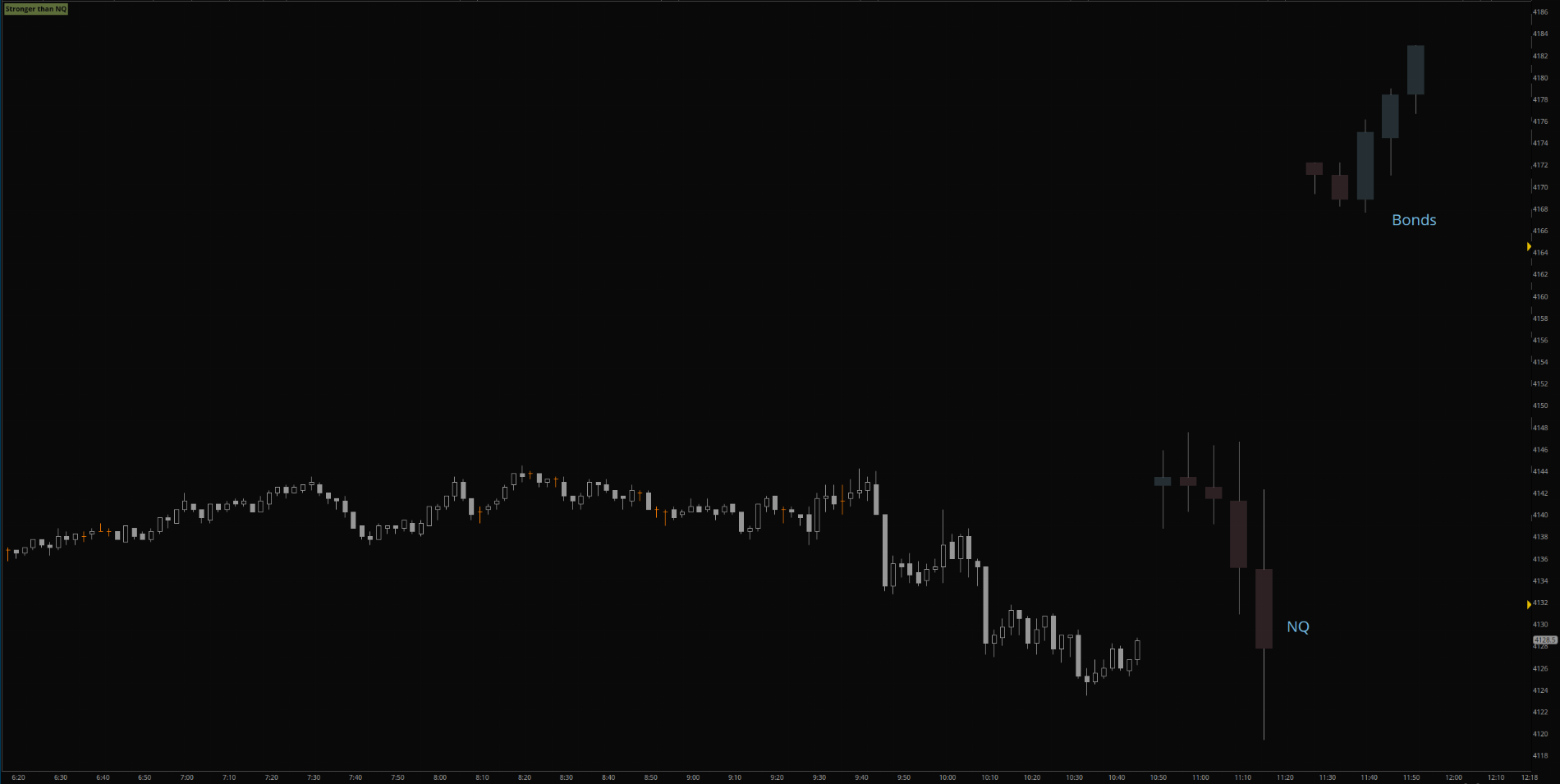•ahramper1 and Fluideng

This is simply a tool for price action traders. Showing you a higher timeframe candle (or candles) on a smaller timeframe chart. Helping you keep perspective of the larger move.

•ahramper1

### Not the exact question you're looking for?

87k+ Posts
387 Online## The Market Trading Game Changer

Join 2,500+ subscribers inside the useThinkScript VIP Membership Club
• Exclusive indicators
• Proven strategies & setups
• Private Discord community
• Exclusive members-only content
• 1 full year of unlimited support

What is useThinkScript?

useThinkScript is the #1 community of stock market investors using indicators and other tools to power their trading strategies. Traders of all skill levels use our forums to learn about scripting and indicators, help each other, and discover new ways to gain an edge in the markets.

How do I get started?

We get it. Our forum can be intimidating, if not overwhelming. With thousands of topics, tens of thousands of posts, our community has created an incredibly deep knowledge base for stock traders. No one can ever exhaust every resource provided on our site.

If you are new, or just looking for guidance, here are some helpful links to get you started.

What are the benefits of VIP Membership?